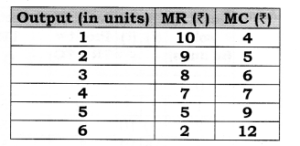# Chapter 9 – Producer Equilibrium Questions and Answers: NCERT Solutions for Class 12 Micro Economics

Class 12 Introduction to Economics NCERT book solutions for Chapter 9 - Producer Equilibrium Questions and Answers.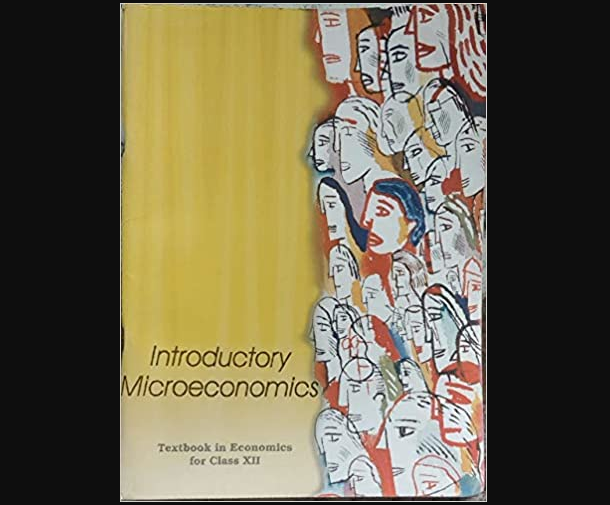### Answer: The conditions must hold if a profit maximizing firm produces positive output in a competitive market when price is constant’under MR/MC approach is determined where, (i) MR = MC (ii) MC must be rising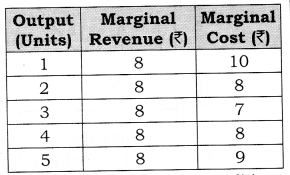### According to Table, both the conditions of equilibrium are satisfied at 4 units of output. MC is equal to MR and MC is rising. MC is more than MR when output is produced after 4 units of output. So, Producer’s Equilibrium will be achieved at 4 units of output. However, MR is equal to MC at 2 units of output also. But, second condition is not fulfilled here. Let us understand the determination of equilibrium with the help of a diagram: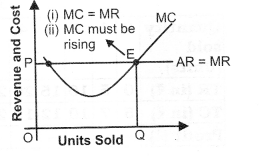## Question 2. Can there be a positive level of output that a profit-maximizing firm produces in a competitive market at which market price is not equal to marginal cost? Give an explanation. Or Explain why will a producer not be in equilibrium if the conditions of equilibrium are not met.

### Answer: The profit maximizing level of output is always determined where, (i) MR = MC (ii) MC must be rising. In other words, where price is equal to MC. If price is not equal to MC, profit maximizing condition cannot hold. It can be explained with the help of the following two cases: Case 1: Price Greater Than MC (i) In the given figure at output level q2, the market price is greater than marginal cost. (ii) To show that q2 is not a profit maximizing level of output, we have taken q3 output level, which is right of qr (iii) Suppose the firm increases its output level from q2 to qr The increase in total revenue of the firm from this output is the market price multiplied by the change in quantity (ATR = market price x AQ), that is, the area of rectangle q2q3CB. (iv) On the other hand, the increase in total cost with this, increase in output is the area of the region q2q3XW. (v) But, a comparison of the two area shows that the firm’s profit is higher when output level is q3 rather than q1 So, q2 is not a profit maximizing level of output. Case 2: Price Less Than MC (i) In the given figure at output level q2, the market price is less than marginal cost. (ii) To show that q2 is not a profit maximizing level of output, we have taken q3 output level, which is left of qr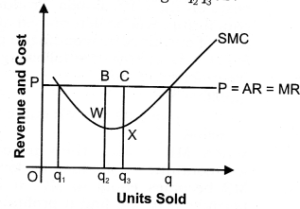### (iii) Suppose now, that the firm reduce its output level from q2 to q1 The decrease in total revenue of the firm from this output is the market price multiplied by the change in quantity (ATR = market price x AQ), that is, the area of rectangle q2q3CB.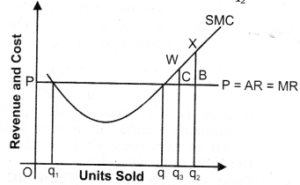## Question 3. Will a profit-maximizing firm in a competitive market ever produce a positive level of output in the range where the marginal cost is falling? Give an explanation.

### Answer: No, as sufficient condition of producer equilibrium is Marginal Cost must be rising when Marginal Cost = Marginal revenue. It can be explained with the help of following diagram: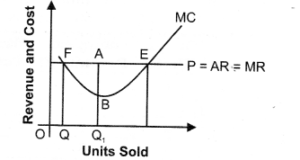## Question 4. The following table shows the total revenue and total cost schedules of a competitive firm. Calculate the profit at each output level. Determine also the market price of the goods.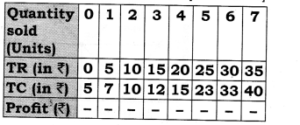### Market price (AR) = TR/Quantity sold = Rs. 5. (TR = Total Revenue; TC = Total Cost)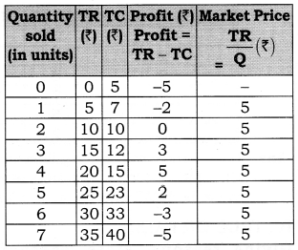## Question 5. The following table shows the total cost schedule of a competitive firm. It is given that the price of the goods is Rs. 10. Calculate the profit at each output level. Find the profit maximising level of output.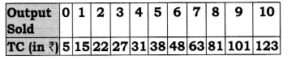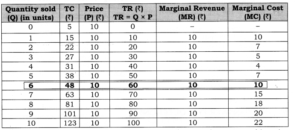## Question 3. Find out the maximum profit position of a producer by comparing his MC and MR on the basis of the following data: